# Get the asymptotic distribution of the estimators¶

In this example we introduce the buildEstimator method to obtain the asymptotic distribution of the parameters of a fitted distribution obtained from a Factory.

import openturns as ot
import openturns.viewer as viewer
from matplotlib import pylab as plt

ot.Log.Show(ot.Log.NONE)


Set the random generator seed

ot.RandomGenerator.SetSeed(0)


## The standard normal¶

The parameters of the standard normal distribution are estimated by a method of moments method. Thus the asymptotic parameters distribution is normal and estimated by bootstrap on the initial data.

distribution = ot.Normal(0.0, 1.0)
sample = distribution.getSample(50)
estimated = ot.NormalFactory().build(sample)


We take a look at the estimated parameters :

print(estimated.getParameter())

[0.0353171,0.968336]


The buildEstimator method gives the asymptotic parameters distribution.

fittedRes = ot.NormalFactory().buildEstimator(sample)
paramDist = fittedRes.getParameterDistribution()


We draw the 2D-PDF of the parameters

graph = paramDist.drawPDF()
graph.setXTitle(r"$\mu$")
graph.setYTitle(r"$\sigma$")
graph.setTitle(r"Normal fitting : $(\mu, \sigma)$ iso-PDF")
view = viewer.View(graph)We draw the mean parameter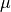distribution

graph = paramDist.getMarginal(0).drawPDF()
graph.setTitle(r"Normal fitting : PDF of $\mu$")
graph.setXTitle(r"$\mu$")
graph.setLegends(["PDF"])
view = viewer.View(graph)We draw the scale parameter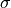distribution

graph = paramDist.getMarginal(1).drawPDF()
graph.setTitle(r"Normal fitting : PDF of $\sigma$")
graph.setXTitle(r"$\sigma$")
graph.setLegends(["PDF"])
view = viewer.View(graph)We observe on the two previous figures that the distribution is normal and centered around the estimated value of the parameter.

## The Pareto distribution¶

We consider a Pareto distribution with a scale parameter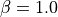, a shape parameter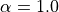and a location parameter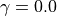. We generate a sample from this distribution and use a ParetoFactory to fit the sample. In that case the asymptotic parameters distribution is estimated by bootstrap on the initial data and kernel fitting (see KernelSmoothing).

distribution = ot.Pareto(1.0, 1.0, 0.0)
sample = distribution.getSample(50)
estimated = ot.ParetoFactory().build(sample)


We take a look at the estimated parameters :

print(estimated.getParameter())

[0.39768,0.696349,0.690277]


The buildEstimator method gives the asymptotic parameters distribution.

fittedRes = ot.ParetoFactory().buildEstimator(sample)
paramDist = fittedRes.getParameterDistribution()


We draw scale parameter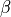distribution

graph = paramDist.getMarginal(0).drawPDF()
graph.setTitle(r"Pareto fitting : PDF of $\beta$")
graph.setXTitle(r"$\beta$")
graph.setLegends(["PDF"])
view = viewer.View(graph)We draw the shape parameter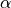distribution

graph = paramDist.getMarginal(1).drawPDF()
graph.setTitle(r"Pareto fitting : PDF of $\alpha$")
graph.setXTitle(r"$\alpha$")
graph.setLegends(["PDF"])
view = viewer.View(graph)We draw the location parameter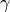distribution

graph = paramDist.getMarginal(2).drawPDF()
graph.setTitle(r"Pareto fitting : PDF of $\gamma$")
graph.setXTitle(r"$\gamma$")
graph.setLegends(["PDF"])
view = viewer.View(graph)

plt.show()